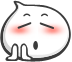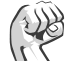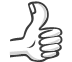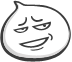### Aspose.Cells小技巧

`sheet.Cells.InsertRows(2, 10);`

`sheet.Cells.DeleteRows(7, 5);`

`cells.Merge(1, 2, 3, 4);`

```sheet.Cells[5, 5].Formula="=A1*B1";
sheet.Cells[5, 5].Formula="=SUM(A1:B1)";
CurrentWorkbook.CalculateFormula(true);```### 鼓掌

0### 正能量

0### 赞

0### 呵呵

0

##### 企业级大促